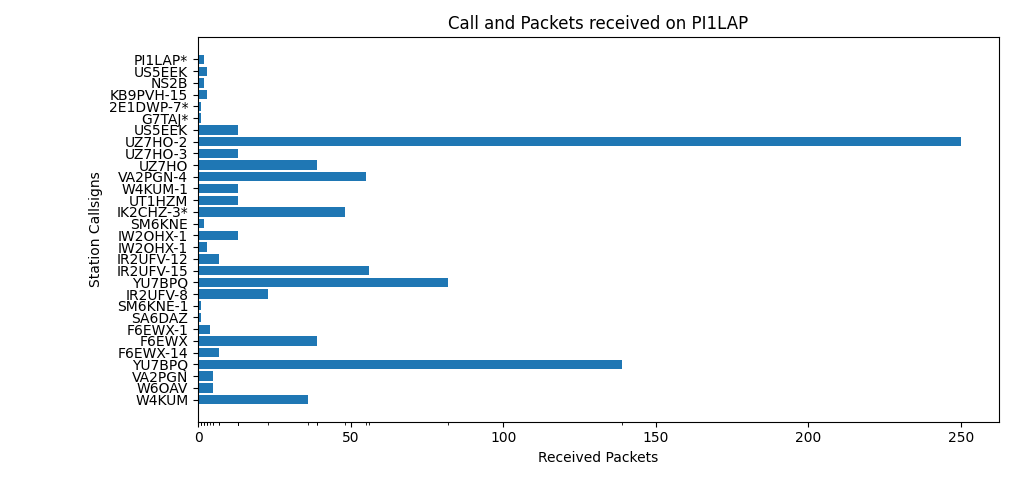## MHSave and PythonToday I am playing with Python and Matplotlib. In BPQ32 you have the option to save the Most Heard list (MH) when you close BPQ32. This contains a lot of data that you can use. Above I made a plot of the received stations and the number of packets I received at 14.1022Mhz + 1000Hz at 300Baud. Now I am not a world programmer, so this could probably be easier or different. Below the script I have written and used.

```#!/usr/bin/python3
import re, csv, zlib
import matplotlib.pyplot as plt
import numpy as np

#First get the data form the right port. 14.1022Mhz 300 Baud HF Packet

port4 = []

with open("MHSave.txt") as f:
found_port4 = False

for line in f:
if line.startswith("Port:4"):

found_port4 = True

if found_port4:
if line.startswith("Port:5"):
break
else:
mod_string = line.replace('||', '')
mod_strings = re.sub("via.*\$", "", mod_string)
port4.append(mod_strings.rstrip('\n'))

with open('port4.txt', 'w') as fout:
fout.writelines("\n".join(port4[1:]))

#print("\n".join(port4))

# Convert it to a csv file
with open('port4.txt', 'r') as in_file:
stripped = [line.replace(","," ").split() for line in lines]
grouped = zip(*[stripped]*1)
with open('port4.csv', 'w') as out_file:
writer = csv.writer(out_file)
writer.writerow(('Epoch', 'Pack-rev', 'Call', 'Month', 'Day', 'Time'))
for group in grouped:
writer.writerows(group)

# Matplotlib create a nice Plot of the received calls

filename = 'port4.csv'
with open(filename) as f:

packs, calls = [], []
pack = int(row)
call = str(row)
packs.append(pack)
calls.append(call)

x = np.arange(len(calls))

fig, ax = plt.subplots()

ax.barh(x, packs, align='center')
ax.set_title("Call and Packets received on PI1LAP")